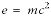# inlineequation

\$Revision: 1666 \$

\$Date: 2002-06-12 07:19:37 -0400 (Wed, 12 Jun 2002) \$

inlineequation — A mathematical equation or expression occurring inline

## Synopsis

### Content Model

```inlineequation ::=
((`alt`?,
(`graphic`+|`inlinemediaobject`+|`mathphrase`+)))```

### Attributes

Common attributes

## Description

`InlineEquation`s are expressions (usually mathematical) that occur in the text flow.

### Processing expectations

Formatted inline.

`InlineEquation` should not contain `Graphic`. Instead, it should contain `InlineGraphic`. Within an `InlineEquation`, `Graphic` should be rendered inline.

### Future Changes

In DocBook V5.0, `InlineGraphic` and `Graphic` will be discarded.

### Children

The following elements occur in inlineequation: .

## Examples

```<!DOCTYPE para PUBLIC "-//OASIS//DTD DocBook XML V4.1.2//EN"
"http://www.oasis-open.org/docbook/xml/4.1.2/docbookx.dtd">
<para>
Einstein's theory of relativity includes one of the most
widely recognized formulas in the world:
<inlineequation>
<alt>e=mc^2</alt>
<graphic fileref="figures/emc2.png"/>
</inlineequation>
</para>```

Einstein's theory of relativity includes one of the most widely recognized formulas in the world:For additional examples, see also `inlinemediaobject`.